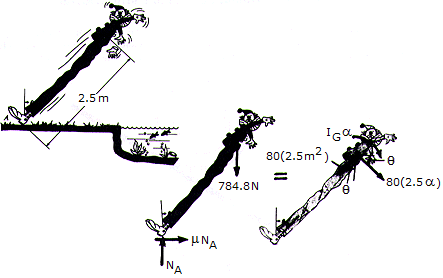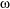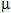# Engineering Mechanics - PKRB: Force and Animation - Discussion

### Discussion :: PKRB: Force and Animation - General Questions (Q.No.2)

2.A clown, mounted on stilts, loses his balance and falls backward from the position, where it is assumed the= 0 when= 07deg;. Paralyzed with fear, he remains rigid as he falls. His mass including the stilts is 80 kg, the mass center is at G, and the radius of gyration about G is kG = 1.2 m. Determine the coefficient of friction between his shoes and the ground at A if it is observed that slipping occurs when= 30°.

 [A].= 0.833 [B].= 0.468 [C].= 0.243 [D].= 0.400

Explanation:

No answer description available for this question.

 Radhika said: (Jun 6, 2011) We know that, F=coefficient of friction * R R= normal force = (80*2.5*9.81) =1962 N F = Force = (80*9.81) N hence, coefficient of friction = F/R = (80*9.81)/(80*2.5*9.81) = 0.4 ans